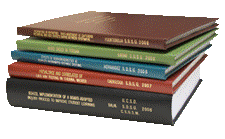Archival Master’s Theses from the University of Nebraska-Lincoln

Thesis

7-1957

Citation

Thesis (M.S.)—University of Nebraska—Lincoln, 1957. Department of Chemistry and Chemical Engineering.

Copyright 1957, the author. Used by permission.

Abstract

The purpose of this study is to evaluate the effects of acid concentration and flow rate past the metal surface on the rate of the continuous dissolution of copper in nitric acid.

The following conclusions were drawn from this study:

(1) The continuous dissolution of electrolytic copper by nitric acid was carried out experimentally at essentially isothermal conditions (29C.) to determine the effects of changes in acid concentration and acid mass velocity.

(2) Increased acid concentration, from 1 N to 5 N, increased the rate of dissolution with a particularly sharp increase occurring between 3 N to 4 N. At a number of mass velocities the analytical expressions for the rate of dissolution per unit area per unit time have been found as a function of acid concentration and the degree of dissociation.

(3) The rate of dissolution was either decreased or not affected appreciably by increasing the acid mass velocity between 7 and 19 gm/sec-cm2. The rates of dissolution per unit area per unit time have been expressed as mathematical functions of the mass velocity at several different acid concentrations.